| my account | login-logout | resources | support | catalog | home | get webcard |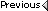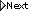» Online Classroom   » Inland and Coastal Navigation   » Public Discussion of Inland and Coastal Navigation   » Middle Latitude Sailing

Author Topic: Middle Latitude Sailing
 David McCarty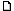posted March 24, 2004 01:30 PM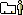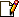I recently came across a WWII navigation and gunnery text which I have found helpful in delving more deeply into the subjects of middle latitude sailing and great circle navigation. Im stumped on a seemingly simple problem however. Perhaps someone out there can help.Consider triangle PNP:1. PN represents a meridian.2. NP represents part of a parallel of latitude.3. PP represents a rhumb line which cuts meridian PN at angle C.4. PP = d = distance.5. PN = l = difference in latitude.6. NP = p = departure.The following formulas are derived in the text (where difference in latitude and difference in longitude are measured in minutes and d is measured in nautical miles): Diff in Lat = d cos C Diff in Long = d sin C sec (1/2)(Lat P + Lat P)Use the above formulas to solve the following problem: Point P = 43 deg 19 min N, 17 deg 42 min W Point P = 41 deg 13 min N, 21 deg 14 min W Find the course and distance for a rhumb line track from point P to point P'.The answer given is: C = 231.2 degrees d = 201.1 nmI dont seem to have enough information to solve the problem. Am I overlooking something? Can you recommend a good current book on the subjects of middle latitude sailing and great circle navigation that goes into more detail than does Bowditch?
 David Burchposted April 04, 2004 11:53 PMSorry i missed this note and no one else jumped in. A rhumbline compuation to your problem gives these as the exact answers: 231.3° and 201.65 nmi. We can, however, get good approximations to this with mid latitude sailing as you ahve presented it.Usually, though, one might use mid lat sailing for figuring a new DR based on C and d from a given position. You pose here the reverse of that, which can be solved with the equations you have, but generally it might be easier to use meridonal parts tables from Bowditch.for your problem: figure dLat = 126' and dLon = 212'.then you have from equ(1) 126 = d x cos(C)for equ(2) you need the mid lat = 42° 16' = 42.2666°, and then you need the cos of that [sec is just 1/cos] and cos (42.66666) = 0.7400so equ(2) can be rewritten as 0.7400 x 212 = d x sin(C) or equ(2) is now 156.88 = d x sin (C).next divide equ(2) by equ(1) to get 1.245 = tan(C)then take arctan function from a calculator to get C = 51.23°, but this is just the angle with the meridian, not the actual course, so you need to add 180° to get C = 231.23° T.now go back to equ(1) to get; 126 = d x cos(51.23) or d = 126/0.6262 = 201.21.These days this type of problem is best solved with programmed calculators such as the StarPilot, which will compute anything you could want in this regard to a higher precsion than you can do it by hand. Indeed, this mid lat approach will break down for larger distances, and also it assumes a spherical earth. If you actually want real distances along a real earth, then it is best to use ellipsoidal computations and insert the earth shape you choose. We have a free program for doing that as a download in the resources section.Again mid lat computations of this type are usually done for figuring a new DR postion based on course and distance run for a short run, or in some cases of cel nav where you just need dLon to figure a time correction.Short of the StarPilot, you can also download the set of programs we have called NIMA calculators and it has a real rhumbline function as well. Note that a proper rhumbline computation is more accurate than the mid latitude sailing but it is also a much more complex formula, which in turn is why one uses the meridonal parts tables for this if you do not have a computer or calculator program.--david From: Starpath, Seattle, WA

 All times are Pacific